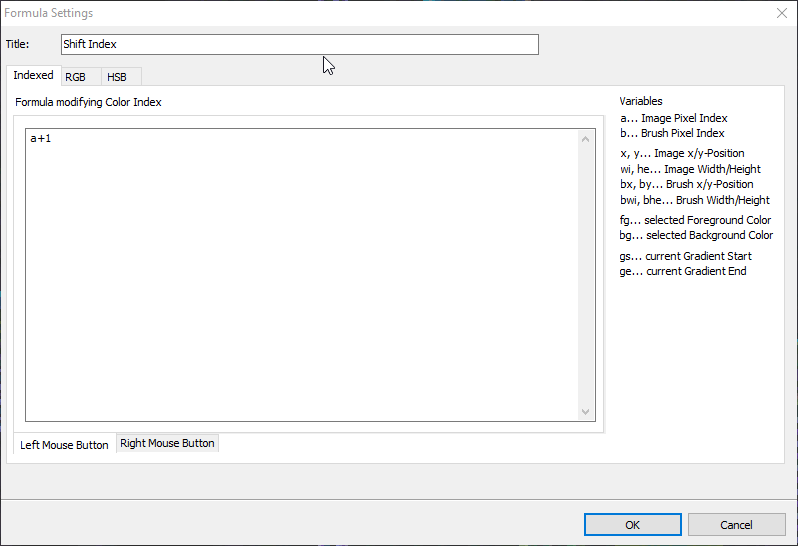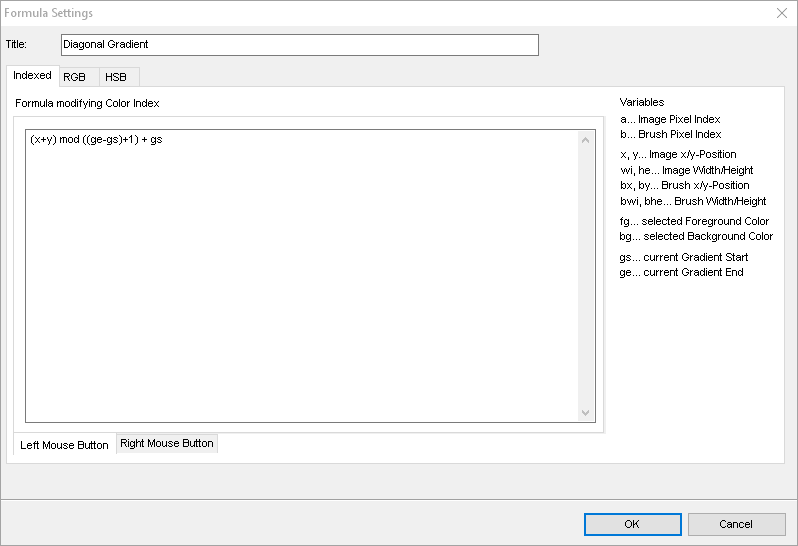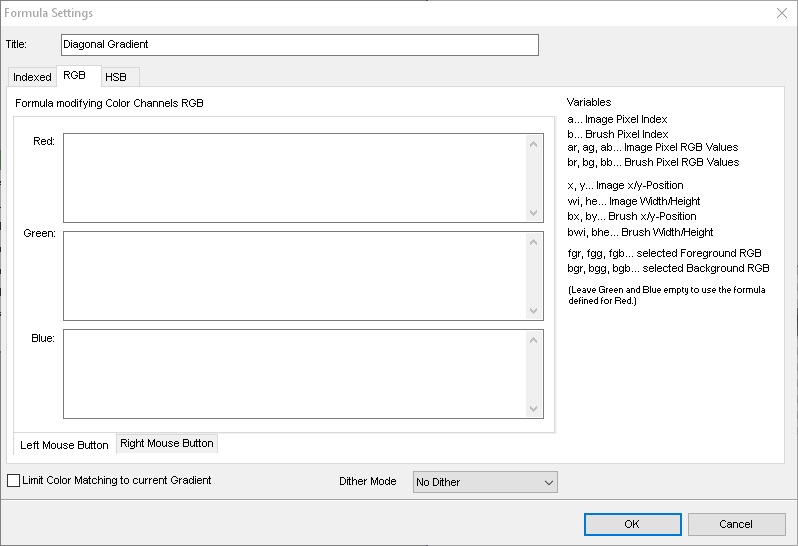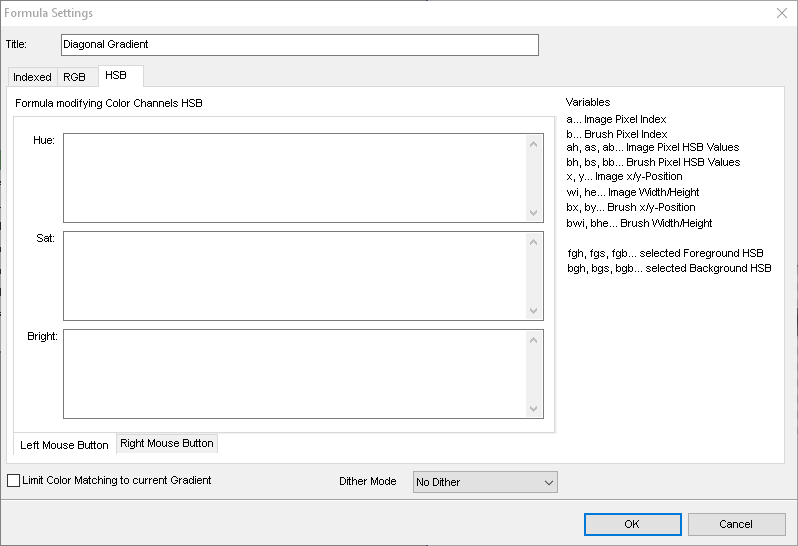# Formula Editor

When you draw with your brush or use filled shapes then the currently selected paint mode is used to modify the pixels on the canvas. Behind every paint mode there is a special logic that combines the pixels on the canvas with the selected drawing color or brush pixels, when you draw with a brush. The paint mode Formula allows you to create your own logics to be applied. That means you can define mathematical formulas or simple logic to fit your needs or to extend the paint modes with new ways of modifying pixels.
When the paint mode Formula is active then you can choose from a range of predefined formulas or add your own in the Paint Mode Settings. This editor is used to define such mathematical formulas based on a set of operators and functions.

Calculations that are to be used as paint mode transformation can be made based on combining color indexes or by doing calculations in RGB or HSB spaces. When applying the formula paint mode Pro Motion passes pixel data of the underlying image and the brush shape (if used) to the corresponding formula. The formula must calculate the new pixel value based on that input.

Before we have a more detailed look at all the possible inputs and operations let's assume a simple example:
When we draw with the left mouse button then we want to make all pixels to shift their palette index up one time. The required formula would be just a + 1 and look like this in the dialog...Take the image pixel index that is to be processed which is just a and increase it by one. It's as simple as that. As said before you can also do calculations in RGB or HSB space which uses color space data as input and output, and you can also define different operations for left or right mouse button usage, and we will go into these detail later. First let's have a look at the mathematical operations that are built in.

These operations can be applied by using operator characters or words. The operations can be calculative (addition, subtraction etc.) or logical (and, or, xor etc.). They all have a predefined priority as shown in the table so that you don't have to always set braces to ensure a priority. Typical priorities you already know from school is that division or multiplication is always calculated before subtraction or addition.

Operator Meaning Priority
and logical and 1
or logical or 1
xor logical xor 1
<= less or equal 2
>= greater or equal 2
!= not equal 2
== equal 2
> greater than 2
< less than 2
- subtraction 3
* multiplication 4
/ division 4
^ raise x to the power of y 5
mod modulus 0

The smaller the priority the later the operator is applied to its operands. As outlined before as example x * 3 - 2 will calculate x * 3 before subtracting 2. To have other priorities you must use braces, like x * (3-2).

Especially all the logic operators that do not calculate something but compare values might seem weird to people not having experience with mathematical logic. It's beyond the scope to describe those operators deeper.

Besides operators there are also built in functions that can be used. In practice only some of them are important, especially if, min, max but if you are a math geek you could also derive effects with all the trigonometry operations. We will use some functions in samples later. Just as a reference we list them all here.

Name # of Arguments Explanation
sin 1 sine function
cos 1 cosine function
tan 1 tangens function
asin 1 arcus sine function
acos 1 arcus cosine function
atan 1 arcus tangens function
sinh 1 hyperbolic sine function
cosh 1 hyperbolic cosine
tanh 1 hyperbolic tangens function
asinh 1 hyperbolic arcus sine function
acosh 1 hyperbolic arcus tangens function
atanh 1 hyperbolic arcur tangens function
log2 1 logarithm to the base 2
log10 1 logarithm to the base 10
log 1 logarithm to the base 10
ln 1 logarithm to base e (2.71828...)
exp 1 e raised to the power of x
sqrt 1 square root of a value
sign 1 sign function -1 if x<0; 1 if x>0
rint 1 round to nearest integer
abs 1 absolute value
if 3 if ... then ... else ... like if(condition, valueIfTrue, valueIfFalse)
min variable min of all arguments
max variable max of all arguments
sum variable sum of all arguments
avg variable mean value of all arguments

The arguments must be passed in braces, e.g. sin(x) or if( x > 2, 7, 6 ). The number of arguments may be variable, for example when using the sum function sum(1, 3, 5, -4).

Let's now have a look at the different sections where you can define formulas for. As mentioned before they are divided into different areas depending on the value space you want to use (color indexes or RGB/HSB color space).
Each section defines the variables that are available when doing the calculation, and you will find them on the right side in the editor. When using the paint mode the program just goes through every pixel on the canvas that is affected, processes the formula and replaces the canvas pixel with the formula result. When you use the brush for painting then consider the brush being placed on the canvas and for every pixel to be processed you have a canvas pixel and a brush pixel that is on top of it. The program iterates through canvas and brush pixels alike. If you use a tool like a filled rectangle or if your brush is single colored like a simple brush tip then the first or second drawing color is used depending on the mouse button you use for drawing.

## Indexed

Calculations working on color indices will get color indices as input data. You can not access any RGB values of the colors.See the variables on the right as a reference. A more detailed description:

Name Explanation
a The color index of the canvas pixel to be processed.
b The color index of the brush pixel to be processed or the selected drawing color.
x, y The current position on the canvas starting with x = 0, y = 0 at the top left of the canvas
wi, he The overall size of the canvas
bx, by The current position within the brush shape, starting with bx = 0, by = 0 at the top left of the brush shape
bwi, bhe The overall size of the brush shape
fg Selected first color
bg Selected second color
gs First palette index of the currently selected gradient
ge Last palette index of the currently selected gradient

You can define formulas with these variables and all the operators and functions listed above. Optionally define different formulas for when using Left Mouse Button or Right Mouse Button. Let's see some examples.

Only draw the first color on those pixels that are not equal to the currently selected second color (default formula Exclusive):
if( a != bg, fg, a )
Translated to text it means "if canvas-pixel is not equal to second-color then the result is first-color or the canvas-pixels itself otherwise".

Skip every second pixel scan line (default formula Scanline):
if( (y mod 2) == 1, a, b )
Translated to text it means "if canvas-y-position can not be divided by 2 then leave the canvas-pixel otherwise apply the brush pixel".

## RGB

Calculations working on RGB color space get RGB values as input data. For the RGB result the best matching color in the color palette is used. The result can be very poor, if the colors in palette differ too much from the results. To improve this you can Limit the Color Matching to current Gradient which will only use best matching colors that can be found in the currently selected gradient or use a Dither Mode.The variables differ concerning pixel data compared to the indexed mode. There are additional variables to carry the RGB values of pixels instead of their indices.

Name Explanation
ar, ag, ab The RGB color values of the canvas pixel to be processed.
br, bg, bb The RGB color value of the brush pixel to be processed or the selected drawing color.
fgr, fgg, fgb Selected first color RGB values
bgr, bgg, bgb Selected second color RGB values

The formulas are to be created the same way as with indexed mode except that you need to define a separate formula for every RGB color channel, meaning one to calculate red, green and blue respectively. You can omit the formulas for green or blue if the same calculation is to be used.

Simple examples...

Turn every pixel to the best matching, average gray value:
Red: max( ar, ag, ab )
Translated to text it means "Use the maximum of the three RGB values for every channel".

Mix brush and canvas colors (50/50):
Red: avg(ar, gr)
Green: avg(ag, bg)
Blue: avg(ab, bb)
Translated to text it means "Use the average between canvas and brush color".

## HSB

Calculations working on HSB color space get HSB values as input data. For the HSB result the best matching color in the color palette is used as mentioned in RGB space. The definitions for color matching are the same as with RGB mode.Just like with the RGB mode the HSB mode does not use color indexes but HSB values per pixel.

Name Explanation
ah, as, ab The HSB color values of the canvas pixel to be processed.
bh, bs, bb The HSB color value of the brush pixel to be processed or the selected drawing color.
fgh, fgs, fgb Selected first color HSB values
bgh, bgs, bgb Selected second color HSB values

Simple example...

Make pixels use the hue of the brush colors:
Hue: bh
Too simple to need an explanation.

The formula parser that is included is the open source "muparser" created by Ingo Berg which can be found at https://beltoforion.de/en/muparser/

## Related topics

Paint Mode Settings